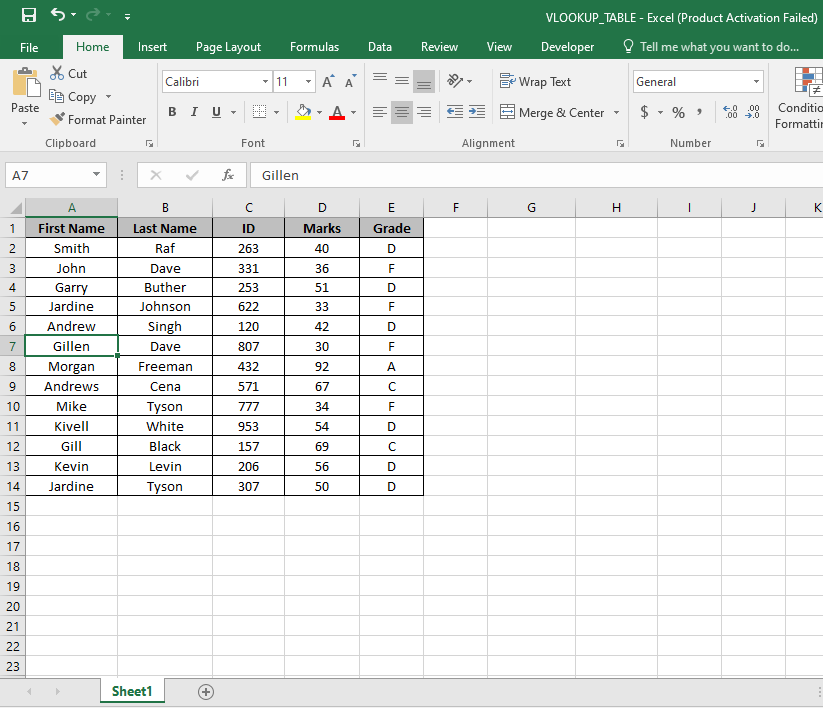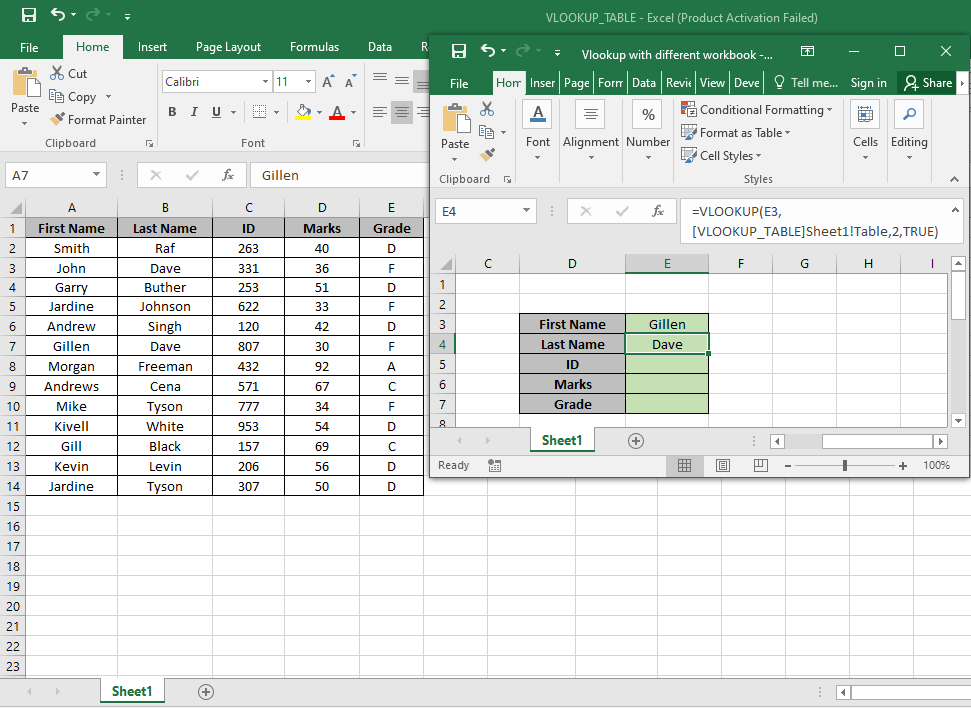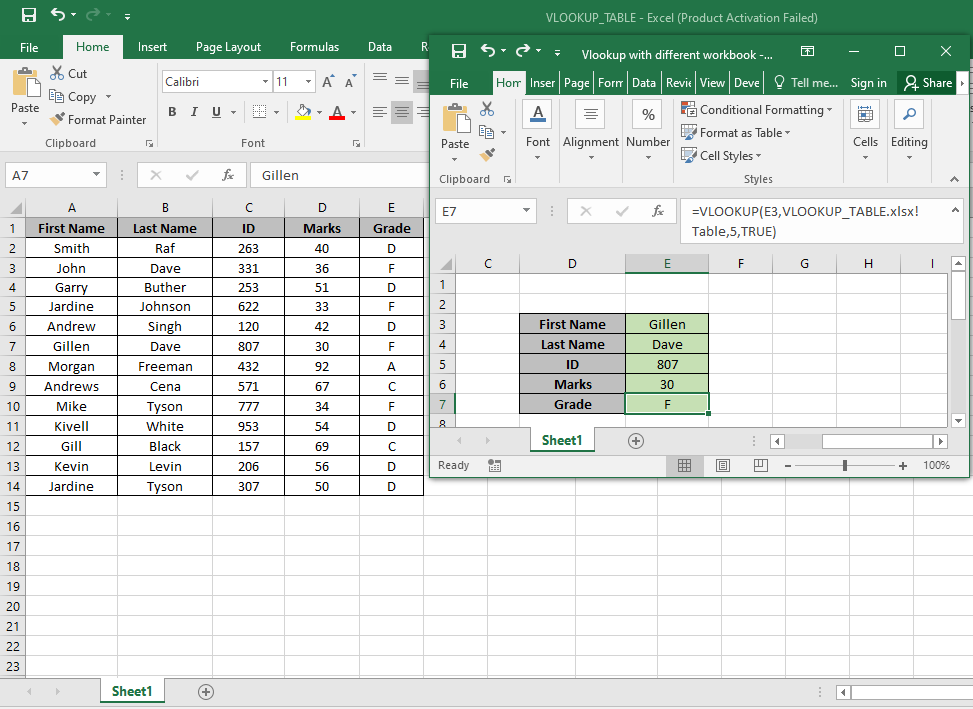# How to use VLOOKUP with Different Excel workbook

First we should understand a little about, What is VLOOKUP function?

VLOOKUP function just searches for the row of a given value in the first column of a table and returns asked column’s value in that row.

Syntax:

= VLOOKUP (lookup_value, table_array, col_index_number, [range_lookup])

Lookup_Value: The Value by which you want to search in the first column of Table Array.
Table_array: The Table in which you want to look up/search
col_index_number: The column number in Table Array from which you want to fetch results.
[range_lookup]: FALSE if you want to search for exact value, TRUE if you want an approximate Date match.

NOTE: VLOOKUP function looks up for the value in the first row of Table_array and extracts corresponding values only right of the selected row data..
To get the value from another workbook table, we just need to apply the simple structure when providing the table_array. Use the below explained structure.

Let’s understand this function using it in an example.

Here we have the table_array in Sheet1 of VLOOKUP_TABLE.xlsx.Here we have a Table named range A2:E14. And we need to find the exact match Gillen with the first name in the Table.
Use the formula:
E3 : Lookup_Value.
[VLOOKUP_TABLE] Sheet1! Table : Complete address of the vlookup table.
2 : look in the Second column of the Table.
TRUE : extracts only exact match.As you can see the first match with Gillen. Now we can find all the corresponding values by the First Name basis

Replace the col_index_number from 2 to 3, 4 & 5 to get the rest of the values.As you can see we got all the values, we needed which matches our exact lookup value.

Notes:

1. The function returns error if workbook address is invalid or wrong.
2. The function returns the error if the value is not matched.

Hope you understood how to use the VLOOKUP with Different Excel workbook in Excel. Explore more articles on Excel HLOOKUP and LOOKUP function here. Please feel free to state your query or feedback for the above article.

Related Articles

How to use the VLOOKUP function in Excel

How to use the HLOOKUP function in Excel

How to VLOOKUP from Different Excel Sheet

Popular Articles

Edit a dropdown list

If with conditional formatting

If with wildcards

Vlookup by date

Terms and Conditions of use

The applications/code on this site are distributed as is and without warranties or liability. In no event shall the owner of the copyrights, or the authors of the applications/code be liable for any loss of profit, any problems or any damage resulting from the use or evaluation of the applications/code.